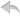注册    登录创作新主题

Python
 python开源   Python   DjangoApp   pycharm   Django
DATA
 docker   Elasticsearch

 分享创造   问与答   求职   闲聊   区块链   招聘   支付之战   翻译   创业   分享发现
WEB开发
 linux   DATABASE   bottle   tornado   NGINX   IE   NoSql   MQ   Bootstrap   js   其他Web框架   Jquery   MongoDB   peewee   web工具   Redis   Git   zookeeper

Python88.com
 反馈   公告   社区推广

Python社区  »  机器学习算法

# R中插入符号机器学习模型的预测值提取

shubhankarnanda • 2 年前 • 244 次点击

``` results<-extractPrediction(models, testX = NULL, testY = NULL, unkX = NULL, unkOnly = !is.null(unkX) & is.null(testX), verbose = FALSE) ```

``` results<-extractPrediction(models) ```

## 在数据集上实现线性支持向量机

``````    cl <- parallel::makeCluster(detectCores(logical=TRUE), type='PSOCK')
doParallel::registerDoParallel(cl)

start.time <- Sys.time()
trnControl <- trainControl(method='cv',number=5, allowParallel = T,verboseIter = T)

set.seed(11)
ds1lnrsvm <- train(shortflag~., data=trnds1,method="svmLinear",
trControl=trnControl)
ds1lnrsvm_t<- Sys.time() - start.time

parallel::stopCluster(cl)
registerDoSEQ()

## Implementing Linear SVM model on test dataset
ds1lnrsvmres <- predict(object=ds1lnrsvm, newdata=testds1)

## confusion matrix to check performance
confusionMatrix(data=ds1lnrsvmres, reference=testds1\$shortflag,positive="1")
``````

Python社区是高质量的Python/Django开发社区•   1 楼 anguyen1210    2 年前 根据你所描述的,一切都应该在 ``` caret ``` . 我解决这个问题的方法是使用 ``` lappy ``` 函数覆盖要估计的所有模型类型的列表。这件事的好处是当你完成后,你将拥有 ``` train ``` 对象整齐地组合在一起,您可以命名这些对象,然后轻松地提取您要查找的任何信息。 现在已经有了这个漂亮的列表,您可以使用另一个apply函数再次遍历它们来创建respective ``` confusionMatrix ``` 对象,您可以将其保存为列表,也可以直接提取与测试集上的预测或准确性相关的任何信息。 从那里,将提取的信息绑定到表中进行打印、比较、绘图等应该非常容易。 例如: ``````# set model names models <- c("plr", "svmLinear", "rpart", "ada", "nnet", "knn") # set train control params trnControl <- trainControl(method='cv',number=5, allowParallel = T,verboseIter = T) # fit models set.seed(11) train_models <- lapply(models, function(model){ print(model) train(shortflag ~ ., method = model, data = trnds1, trControl = trnControl) }) # name the models in your list names(train_models) <- models `````` 有了这个,你有一个很好的列表。如果你这样做了 ``` View(train_models) ``` ,您可以单击并展开所有不同的列表,然后查看可以在其中提取的内容。例如,您可以这样做,以获得训练精度,它返回一个命名列表,以便您知道什么是: ``````# extract training accuracy elapsed <- sapply(train_models, function(object) object\$results["Accuracy"]) `````` 接下来,使用训练过的模型列表,通过创建 ``` 混淆矩阵 ``` 得到你想要的关于测试数据的任何信息。下面是从测试集中直接提取模型精度列表的示例: ``````# extract test accuracy test_accuracy = sapply(train_models, function(x){ pred = predict(x, newdata = testds1) cm = confusionMatrix(pred, reference = testds1\$shortflag) return(cm[["overall"]]["Accuracy"]) `````` 一句忠告:如果你决定尝试这种方法,我会先从你的训练和测试数据中提取一小部分,然后在你开始使用你的所有数据之前,先让代码完全按照你的要求在这个小部分上运行,就像你把整个模型列表输入 ``` lapply ``` 功能如上面第一步所述,可能需要很长时间才能完成。 希望能帮上忙!•   2 楼 cuttlefish44    2 年前 我不能复制你的问题没有 ``` trnds1 ``` / ``` testds1, ``` 但也许下面是你想要的。 ``````library(dplyr) pred <- extractPrediction(list(NAME = ds1lnrsvm), # extractPrediction want list(model) testX = select(testds1, -shortflag), testY = pull(testds1, shortflag)) trnds1 %>% # train data bind_rows(testds1) %>% # bind_rows with testdata bind_cols(pred) # %>% # add cols of predict # select(-shortflag) # shortflg is the same as obs, if you want please delete the col. ``````